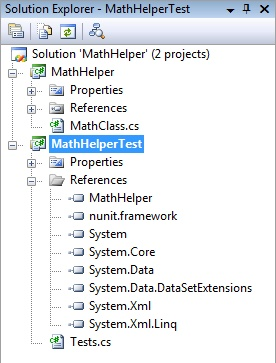### TDD with NUnit

If you haven’t read my first NUnit post and aren’t familiar with NUnit or TDD, you might want to check it out here.

Last time we just looked at the basic ways that you make tests and use the test runner to verify their results. In this post, I’d like to examine how you might go about doing true “Red. Green. Refactor.” Test Driven Development (TDD).

First, I’d like to make a new C# Class Library Application named MathHelper. Rename Class1.cs to MathClass. Additionally, immediately add another project to the solution. Right click on the solution, Add-> New Project. Choose a Class Library Project and call it MathHelperTest. Rename Class1.cs to Tests.cs. Add a reference to the nunit.framework dll and the output from our MathHelper project. Your Solution Explorer should now look like this.Now, we do need to have something so that the test class will compile and we do have a general idea of what we need from requirements gathering, so put the following code in the MathClass.cs file inside the MathHelper project.

```using System;

namespace MathHelper
{
public class MathClass
{
public static double Add(double p1, double p2)
{
throw new NotImplementedException();
}

public static double Subtract(double p1, double p2)
{
throw new NotImplementedException();
}

public static double RaiseToPower(double baseNumber, double exponent)
{
throw new NotImplementedException();
}

public static double Factorial(int number)
{
throw new NotImplementedException();
}
}
}
```

What you can see is that I’ve stubbed in the methods that I expect my class to contain. What I’ve also done is make sure they all throw NotImplementedExceptions when they are called, since I’m not writing any functional code until after I write my tests.

So, lets write some tests already! In Tests.cs inside of our MathHelperTest project, enter the following code.

```using NUnit.Framework;
using MathHelper;

namespace MathHelperTest
{
[TestFixture]
public class Tests
{
[Test]
{
}

[Test]
public void TestStandardSubtract()
{
Assert.AreEqual(31, MathClass.Subtract(77, 46));
}

[Test]
public void TestStandardSquareExponent()
{
Assert.AreEqual(25, MathClass.RaiseToPower(5, 2));
}

[Test]
public void TestStandardFactorial()
{
Assert.AreEqual(120, MathClass.Factorial(5));
}
}
}
```

Okay, for the sake of simplicity, lets fire up our NUnit GUI and load the MathHelperTest.dll and run our test suite. (If you are unsure how to do this, please refer to my initial NUnit post). It should come as no surprise that all four of our tests failed with Not Implemented failures. Now, lets go back and add some code to make the tests pass.

Change the code in MathClass.cs to this:

```using System;

namespace MathHelper
{
public class MathClass
{
public static double Add(double p1, double p2)
{
return p1 + p2;
}

public static double Subtract(double p1, double p2)
{
return p1 - p2;
}

public static double RaiseToPower(double baseNumber, double exponent)
{

for (int i = 0; i < exponent; i++)
{
}

}

public static double Factorial(int number)
{

for (int i = 1; i <= number; i++)
{
}

}
}
}
```

Run the tests again and they should all 4 pass. Woohoo, we’re done, right? Well, not exactly. First of all, our test coverage met the minimum requirements, but didn’t really test the code very well. Secondly, our Math implementation isn’t very great. Let’s solve the first problem first and add some more tests.

```using NUnit.Framework;
using MathHelper;
using System;

namespace MathHelperTest
{
[TestFixture]
public class Tests
{
[Test]
{
}

[Test]
[Category("Subtract Method")]
public void TestStandardSubtract()
{
Assert.AreEqual(31, MathClass.Subtract(77, 46));
}

[Test]
[Category("Exponent Method")]
public void TestStandardSquareExponent()
{
Assert.AreEqual(25, MathClass.RaiseToPower(5, 2));
}

[Test]
[Category("Factorial Method")]
public void TestStandardFactorial()
{
Assert.AreEqual(120, MathClass.Factorial(5));
}

[Test]
{
}

[Test]
[Category("Subtract Method")]
public void TestNegativeSubtract()
{
Assert.AreEqual(90, MathClass.Subtract(45, -45));
}

[Test]
[Category("Exponent Method")]
public void TestNegativeExponent()
{
Assert.AreEqual(.25, MathClass.RaiseToPower(2, -2));
}

[Test]
[Category("Exponent Method")]
public void TestDecimalExponent()
{
Assert.AreEqual(5, MathClass.RaiseToPower(25, .5));
}

}
}
```

When we rerun our tests in the NUnit Test Runner, we now see that two of them fail (TestDecimalExponent and TestNegativeExponent). Lets see if we can fix our code. MathClass.cs should now look like this.

```using System;

namespace MathHelper
{
public class MathClass
{
public static double Add(double p1, double p2)
{
return p1 + p2;
}

public static double Subtract(double p1, double p2)
{
return p1 - p2;
}

public static double RaiseToPower(double baseNumber, double exponent)
{
return Math.Pow(baseNumber, exponent);
}

public static double Factorial(int number)
{

for (int i = 1; i <= number; i++)
{
}

}
}
}
```

Okay, all tests pass. Now, lets see if we can do the Factorial method a little better and use a little recursion. We can safely experiment, because we know that our unit test will ensure that it still returns the correct value.

```        public static double Factorial(int number)
{
// Lets do this recursively.